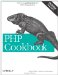# Recipe 3.5. Finding the Difference of Two Dates

#### 3.5.1. Problem

You want to find the elapsed time between two dates. For example, you want to tell a user how long it's been since she last logged onto your site.

#### 3.5.2. Solution

Convert both dates to epoch timestamps and subtract one from the other. Example 3-13 separates the difference into weeks, days, hours, minutes, and seconds.

##### Calculating the difference between two dates

 ``

Example 3-13 prints:

`The two dates have 128 weeks, 6 days, 14 hours, 3 minutes, and 45 seconds elapsed between them.`

Note that the difference isn't divided into larger chunks than weeks (i.e., months or years) because those chunks have variable length and wouldn't give an accurate count of the time difference calculated.

#### 3.5.3. Discussion

There are a few strange things going on here that you should be aware of. First of all, 1962 and 1965 precede the beginning of the epoch. Fortunately, mktime( ) fails gracefully here and produces negative epoch timestamps for each. This is okay because the absolute time value of either of these questionable timestamps isn't necessary, just the difference between the two. As long as epoch timestamps for the dates fall within the range of a signed integer, their difference is calculated correctly.

Next, a wall clock (or calendar) reflects a slightly different amount of time change between these two dates, because they are on different sides of a DST switch. The result subtracting epoch timestamps gives is the correct amount of elapsed time, but the perceived human time change is an hour off. For example, on the Sunday morning in April when DST is activated, what's the difference between 1:30 A.M. and 4:30 A.M.? It seems like three hours, but the epoch timestamps for these two times are only 7,200 seconds apart'two hours. When a local clock springs forward an hour (or falls back an hour in October), the steady march of epoch timestamps takes no notice. Truly, only two hours have passed, although our clock manipulations make it seem like three.

If you want to measure actual elapsed time (and you usually do), this method is fine. If you're more concerned with the difference in what a clock says at two points in time, use Julian days to compute the interval, as discussed in Recipe 3.6.

To tell a user the elapsed time since her last login, you need to find the difference between the login time and her last login time, as shown in Example 3-14.

##### Finding elapsed time since last login

 `prepare("SELECT UNIX_TIMESTAMP(last_login) AS login " . "FROM user WHERE id = ?"); \$st->execute(array(\$id)); \$row = \$st->fetch(); \$epoch_2 = \$row['login']; \$diff_seconds = \$epoch_1 - \$epoch_2; \$diff_weeks = floor(\$diff_seconds/604800); \$diff_seconds -= \$diff_weeks * 604800; \$diff_days = floor(\$diff_seconds/86400); \$diff_seconds -= \$diff_days * 86400; \$diff_hours = floor(\$diff_seconds/3600); \$diff_seconds -= \$diff_hours * 3600; \$diff_minutes = floor(\$diff_seconds/60); \$diff_seconds -= \$diff_minutes * 60; print "You last logged in \$diff_weeks weeks, \$diff_days days, "; print "\$diff_hours hours, \$diff_minutes minutes, and \$diff_seconds ago.";`

Recipe 3.6 to find the difference between two dates with Julian days; Recipe 3.10 for adding to and subtracting from a date; documentation on MySQL's UNIX_TIMESTAMP( ) function can be found at http://www.mysql.com/doc/D/a/Date_and_time_functions.html.PHP Cookbook: Solutions and Examples for PHP Programmers
ISBN: 0596101015
EAN: 2147483647
Year: 2006
Pages: 445

Similar book on Amazon